## BYCOL Function in Google Sheets – LAMBDA Helper Function (New For 2022!)

The BYCOL function in Google Sheets operates on an array or range and returns a new row array, created by grouping each column to a single value.

Here’s a simple example, showing a table of exam scores: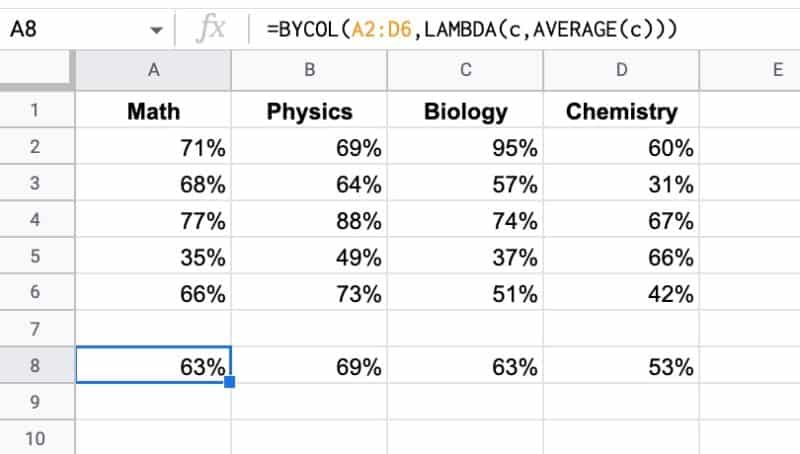The formula in A8 is:

`=BYCOL(A2:D6,LAMBDA(c,AVERAGE(c)))`

The easiest way to think about using BYCOL is to think “what function can I use on a single column?” and then think of BYCOL as just repeating that operation across multiple columns.

BYCOL passes the input array to a lambda function, which takes an input column, called “c”, and calculates the average value for that column. It loops over each column and returns a row array of average values, one for each column.

🔗 Get this example and others in the template at the bottom of this article.

## BYROW Function in Google Sheets – LAMBDA Helper Function (New For 2022!)

The BYROW function in Google Sheets operates on an array or range and returns a new column array, created by grouping each row to a single value.

The value for each row is obtained by applying a lambda function on that row.

For example, this BYROW function calculates the average score of all three rows in the input array:

`=BYROW(A2:D4,LAMBDA(row, AVERAGE(row)))`

Which looks like this in our Google Sheet: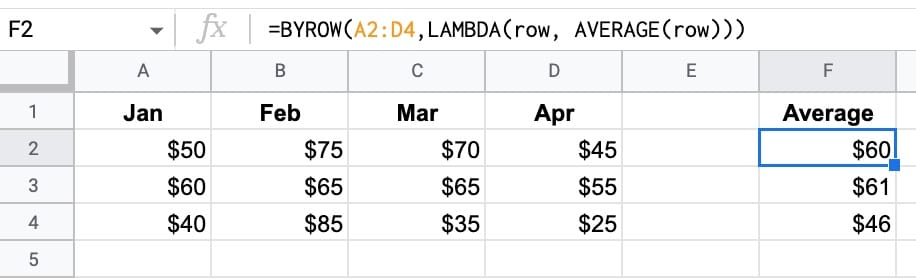(Of course, you could use three separate AVERAGE functions to perform this calculation.)

But the important thing here is how the BYROW operates. It’s much more like programming.

We pass an array of data to the BYROW function.

It then passes each row into a lamba function to calculate a single value for that row (the average in this example). The BYROW formula returns these values in a column array, with the same number of rows as the original input array.

🔗 Get this example and others in the template at the bottom of this article.

## MAKEARRAY Function in Google Sheets – LAMBDA Helper Function (New For 2022!)

The MAKEARRAY function in Google Sheets generates an array of a specified size, with each value calculated by a custom lambda function.

The Lamba function has access to the row and column indices for each value.

Let’s see a simple example:

`=MAKEARRAY(5,3,LAMBDA(row, col, row + col))`

This generates an array with 5 rows and 3 columns.

The value of each element in the row is the sum of the row position and the column position, within the array.

So the first value is 2 (row 1 + column 1) and the final value is 8 (row 5 + column 3). The indices are in relation to the position within the array, not the position within the Google Sheet.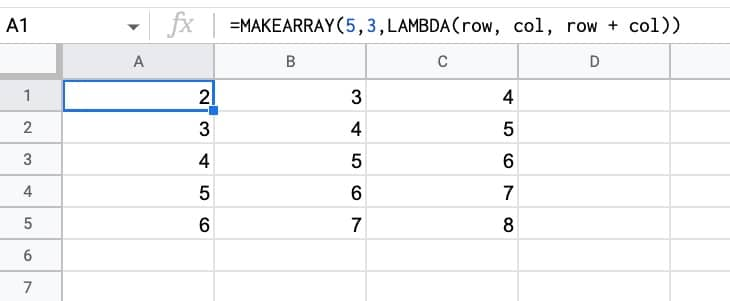🔗 Get this example and others in the template at the bottom of this article.

## SCAN Function in Google Sheets – LAMBDA Helper Function (New For 2022!)

The SCAN function in Google Sheets scans an array and applies a LAMBDA function to each value, moving row by row. The output is an array of intermediate values obtained at each step.

It’s hard to understand without seeing an example, so let’s do that.

The most straightforward SCAN formula is to create a running total calculation on a column of numbers.

The formula is:

`=SCAN(0,A2:A11,LAMBDA(runningTotal,currentValue,runningTotal + currentValue))`

Which produces this array output: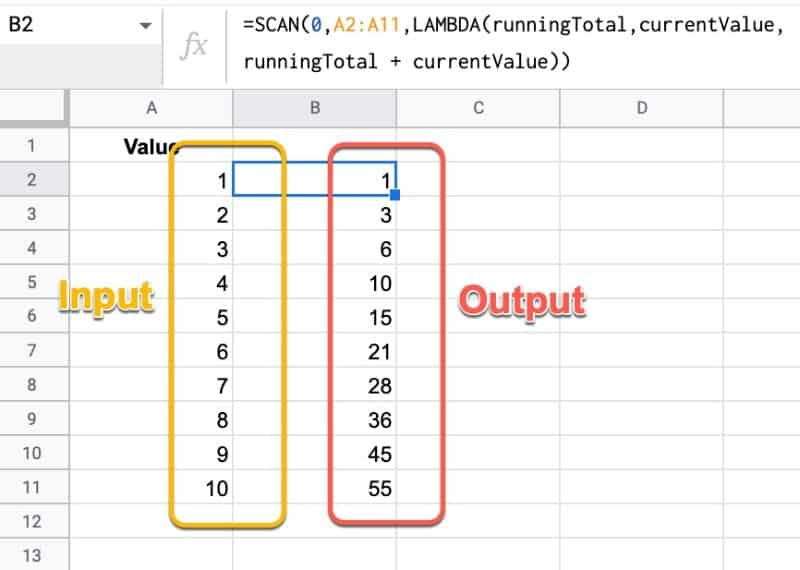The lambda simply adds together the current running total and the new value, then returns the answer to the output array.

When all of the values from the input array have been considered, the output array is returned.

🔗 Get this example and others in the template at the bottom of this article.

## REDUCE Function in Google Sheets – LAMBDA Helper Function (New For 2022!)

The REDUCE function in Google Sheets turns an array input into a single value, by applying a custom LAMBDA function to each value of the input array.

It reduces an array down to a single value.

It’s best to understand through a simple example: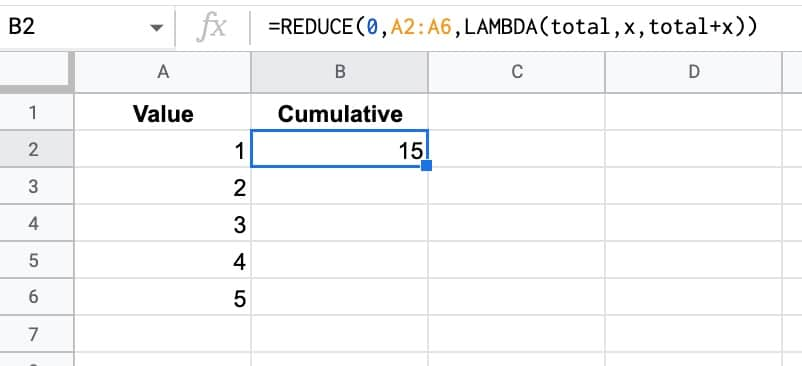The formula in this example is:

`=REDUCE(0,A2:A6,LAMBDA(total,x,total+x))`

The REDUCE function takes a starting value (0 in this example), an input array, and then a custom LAMBDA function.

The formula applies the custom LAMBDA function to each value in the array. In this case, it takes the current total value and adds the next value in the array to it to make a new total. This new total is passed on to the next iteration.

So this REDUCE formula calculates the cumulative total of the 5 numbers.

(And yes, this formula also gets you there:

`=SUM(A2:A6)`

This REDUCE was deliberately simple to show you how it works.)

🔗 Get this example and others in the template at the bottom of this article.